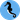# Hippocampus's Garden

Under the sea, in the hippocampus's garden...

# From Direct Method to Doubly Robust

July 29, 2020  |  4 min read  |  71 views

Counterfactual machine learning is drawing more and more attention these days. This research field is now often associated with applications to advertisements and reinforcement learning, but most of its fundamentals were developed in the field of epidemiology as causal inference

In this post, I briefly review three popular techniques developed in causal inference and formulate them with the simple notations used in bandit problems. These techniques are useful for adjusting confounding factors, off-policy evaluation (OPE), completing missing-not-at-randoms (MNARs), etc.

## Notations

Following the customs in contextual bandit, I use these notations and terms in the following part.

Notation Meaning
$\mu$ Behavior (logging) policy
$\pi$ Evaluation policy
$x_i$ Context
$a_i$ Selected arm
$r_i$ Reward
$n$ The number of records in the log
$\{(x_i,a_i,r_i)\}_{i=1}^n$ Log (data) from behavior policy
$\mathcal{A}$ Set of possible arms
$\hat{V}(\pi)$ Estimated value of policy $\pi$

In recommender systems, you can interpret the notation as follows: $x_i$ is a user feature, $a_i$ is a recommended item, $r_i$ is whether the user buy it or not, and $\hat{V}(\pi)$ is the estimated conversion rate (CVR).

## Direct Method

Direct method (DM) simply predicts the counterfactual outcomes. One can train a model with the log from the behavior policy $\mu$ to predict the reward given context and possible arms, and use the predicted reward $\hat{r}(x_i,a)$ to estimate the value of the evaluation policy $\pi$.

$\hat{V}_{\mathrm{DM}}(\pi) = \frac{1}{n}\sum_{i=1}^{n} \sum_{a\in\mathcal{A}}\hat{r}(x_i,a)\pi(a|x_i)$

DM has low variance but its bias is large when the model is mis-specified.

## Importance Sampling

Importance sampling (IS) sums the observed rewards $\{r_i\}_{i=1}^n$, but with weights of probability ratio. That is, it emphasizes the importance of the rewards that happen often in the evaluation policy $\pi$ and rarely in the behavior policy $\mu$.

$\hat{V}_{\mathrm{IS}}(\pi) = \frac{1}{n}\sum_{i=1}^{n} r_i\frac{\pi(a_i|x_i)}{\mu(a_i|x_i)}$

IS is provably unbiased when the behavior policy is known, but it has high variance when the two policies differ a lot, where the weight term is unstable. When the behavior policy is unknown (e.g., observational data), you need to estimate it, and IS is no more unbiased.

IS is also referred to as inverse propensity scoring (IPS).

## Doubly Robust

Doubly robust (DR) combines the above two methods. At first glance, it has a scary-looking definition, but when you compare line 1 and line 2, you will see DR is a combination of DM and residual IS.

\begin{aligned} \hat{V}_{\mathrm{DR}}(\pi) &=& \frac{1}{n}\sum_{i=1}^{n} \Biggl[ (r_i-\hat{r}(x_i,a_i))\frac{\pi(a_i|x_i)}{\mu(a_i|x_i)} + \sum_{a\in\mathcal{A}}\hat{r}(x_i,a)\pi(a|x_i) \Biggr]\\ &=& \hat{V}_{\mathrm{IS}}(\pi) - \frac{1}{n}\sum_{i=1}^{n}\hat{r}(x_i,a_i)\frac{\pi(a_i|x_i)}{\mu(a_i|x_i)} + \hat{V}_{\mathrm{DM}}(\pi) \end{aligned}

DR is unbiased when the DM model is well-specified or the behavior policy is known. In this sense, this method is “doubly robust”. Its variance is lower than IS.

There are some advances like .

 Maria Dimakopoulou. Slate Bandit Learning & Evaluation. 2020.